﻿ Water Resources Planning Institute, WRA, MOEA-Introduction Of Groundwater Simulation Software – FEMWATER
1. ## Introduction

FEMWATER（Three-Dimensional Finite Element Model of Water Flow Through Saturated- Unsaturated Media） was originally written by Dr. Gour-Tsyh Yeh at Pennsylvania State University. A numerical model that simulates the movement of saturated and unsaturated soil moisture in three dimensions. The governing equations for flow are basically the modified Richard equation. This model can solve the problem of saturated, unsaturated, partially saturated unsaturated groundwater flow and density flow pollution transmission. FEMWATER is often used to simulate groundwater flow, contaminant transport and seawater through saturated-unsaturated porous media. One of the widely used groundwater models.

The difference between this mode and the most commonly used MODFLOW is the calculation method. MODFLOW is operated in finite difference mode,and FEMWATER model is operated in a finite element manner. At present, in addition to the FORTRAN program written by Mr. Ye, there are commercial software available.

The FEMWATER analysis steps are shown in Figure 1 to 3. First establish the terrain quality model of the simulation area, shown as Figure 1 , and then build a two-dimensional grid of simulated range, shown as Figure 2, in order to follow the given parameters in the grid. Finally set the simulation range boundary, shown as Figure 3.

2.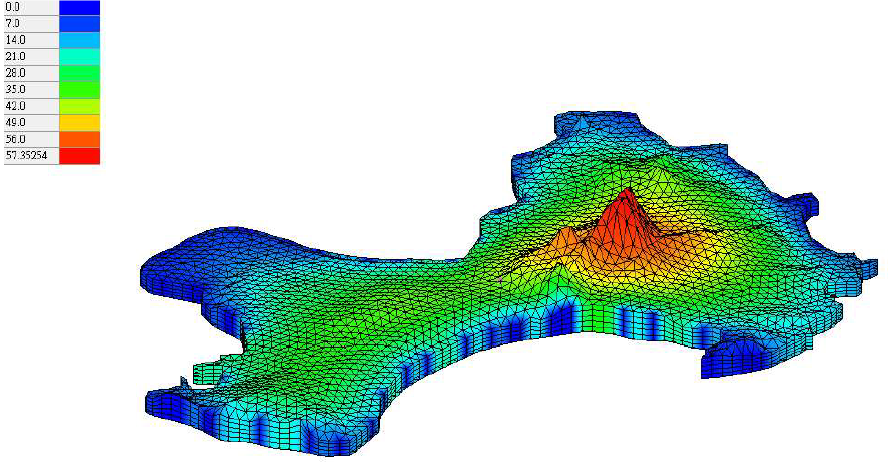Figure.1 DEM model in simulation area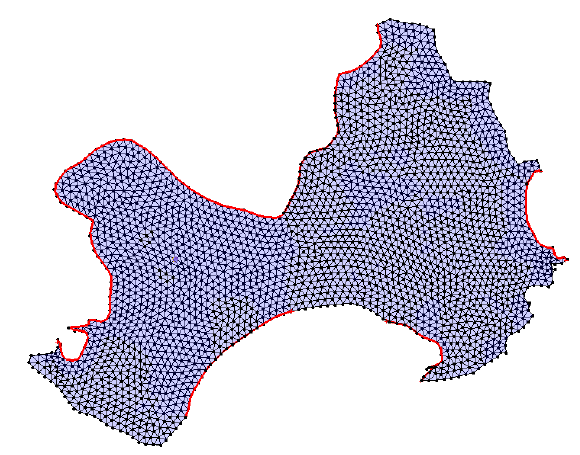Figure.2 Numerical grid in simulation area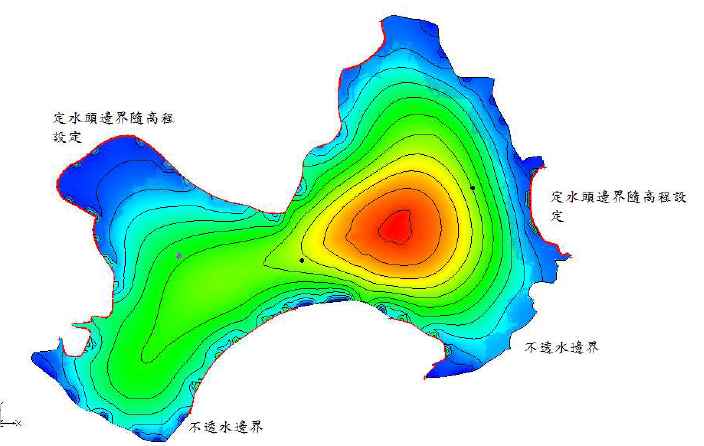Figure.3 Boundary condition in simulation area

3. ## Calculation Equation

FEMWATER mode simulates groundwater problems with finite element method. FEMWATER is often used to simulate groundwater flow, contaminant transport and seawater through saturated-unsaturated porous media. It’s one of the widely used groundwater models. The governing equations for flow are basically the modified Richards equation. For a conservation of fluid and unsaturated layer moisture, and can describe saturated-unsaturated soil media.

1. ### Water flow equation

We can use this equation to simulate groundwater condition after pumping or injecting water. Because the water flow will be changed by water-head.

`ρ/ρ_o (dθ)/(dh) (∂h)/(∂t) = ∇⋅[K(∇h+ρ/ρ_o ∇z)]+ρ^*/ρ_o q`

`ρ` = water density at chemical concentration C

`ρ_o` = referenced water density at zero chemical concentration

`θ` = moisture content

`K` = hydraulic conductivity tensor

`ρ^*` = density of either the injection fluid or the withdrawn water

`q` = source and/or sink

`t` = time

`ρ/ρ_0 (dθ)/(dh)` = storage coefficient

2. ### Concentration diffusion equation

This equation can be used to define the concentration diffusion underground layer. It also can be defined the pollution investigation and remediation.

`θ ∂C/∂t+ρ_b ∂C/∂t+V⋅∇C-∇⋅(θD⋅∇C)=-(α'∂h/∂t+λ)(θC+ρ_b S)-(θK_w C+ρ_b K_s S)``++m-p^*/ρ qC+[F ∂h/∂t+ρ_0/ρ V⋅∇(ρ/ρ_0 )-∂θ/∂t]C` `ρ_b`= bulk density of the medium

`C` = material concentration in aqueous phase

`S` = material concentration in adsorbed phase

`V` = discharge

`D` = dispersion coefficient tensor

`λ` = decay constant

`m` = `qC`in = material concentration in the source

`C`in = material concentration in the source

`K_w` = first order biodegradation rate constant through dissolved phase

3. ### Conclusion

As mentioned above, FEMWATER can be used as groundwater transmission simulation. The simulation results are shown in Figure 4 and Figure 5. Figure 4 is a plot of the simulated water flow direction and the groundwater level. Figure 5 shows the range of influence of water level leakage after simulating pumping behavior.

4.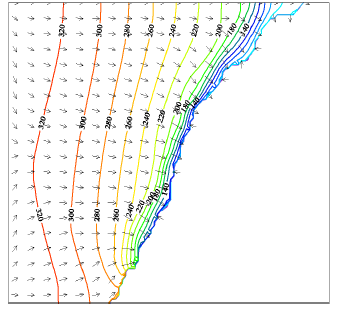Figure.4 water flow simulation conclusion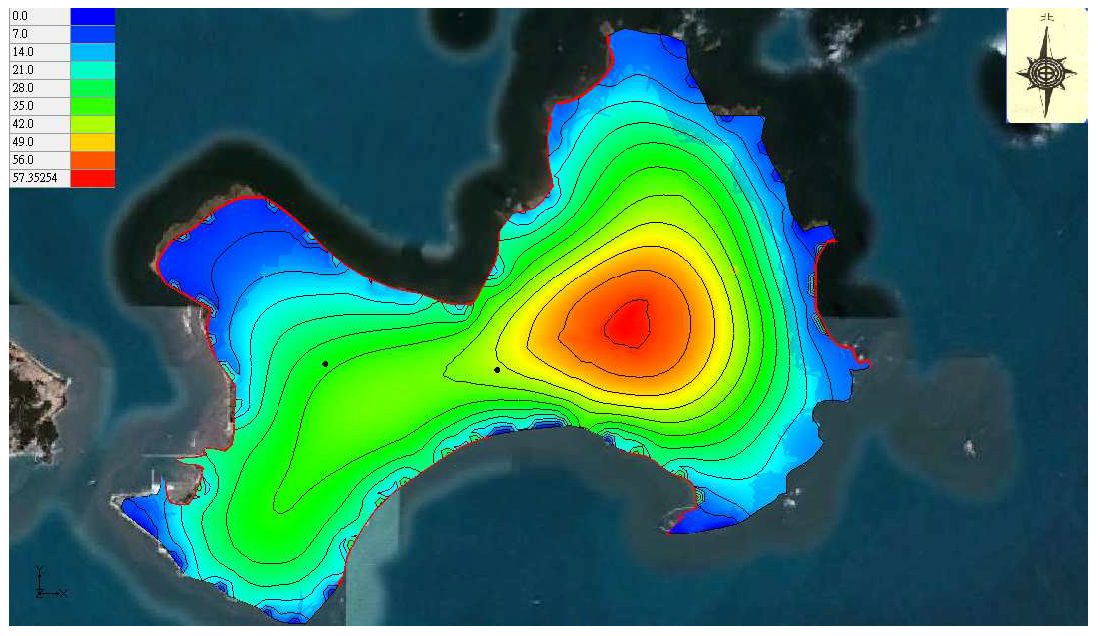Figure.5 groundwater dropdown simulation conclusion

5. ### References

1. Lin, H. C. J., Richards, D. R., Yeh, G. T., Cheng, J. R., & Cheng, H. P. (1997). FEMWATER: A Three-Dimensional Finite Element Computer Model for Simulating Density-Dependent Flow and Transport in Variably Saturated Media (No. WES/TR/CHL-97-12). ARMY ENGINEER WATERWAYS EXPERIMENT STATION VICKSBURG MS COASTAL HYDRAULICS LA B.